Next: Orbital Energies Up: Planetary Motion Previous: Kepler's First Law

# Kepler's Third Law

We have seen that the radius vector connecting our planet to the origin sweeps out area at the constant rate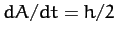[see Equation (249)]. We have also seen that the planetary orbit is an ellipse. Suppose that the major and minor radii of the ellipse are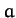and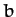, respectively. It follows that the area of the ellipse is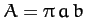. Now, we expect the radius vector to sweep out the whole area of the ellipse in a single orbital period,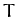. Hence,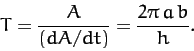(257)

It follows from Equations (236), (237), and (256) that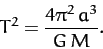(258)

In other words, the square of the orbital period of our planet is proportional to the cube of its orbital major radius--this is Kepler's third law.

Note that for an elliptical orbit the closest distance to the Sun--the so-called perihelion distance--is [see Equations (236) and (255)]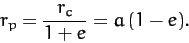(259)

Likewise, the furthest distance from the Sun--the so-called aphelion distance--is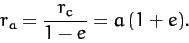(260)

It follows that the major radius,, is simply the mean of the perihelion and aphelion distances,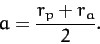(261)

The parameter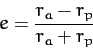(262)

is called the eccentricity, and measures the deviation of the orbit from circularity. Thus,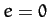corresponds to a circular orbit, whereas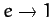corresponds to an infinitely elongated elliptical orbit.

As is easily demonstrated from the above analysis, Kepler laws of planetary motion can be written in the convenient form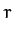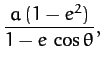(263)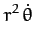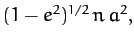(264)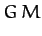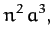(265)

whereis the mean orbital radius (i.e., the major radius),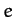the orbital eccentricity, and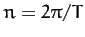the mean orbital angular velocity.Next: Orbital Energies Up: Planetary Motion Previous: Kepler's First Law
Richard Fitzpatrick 2011-03-31